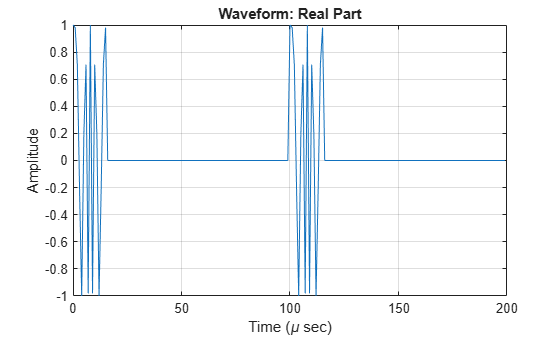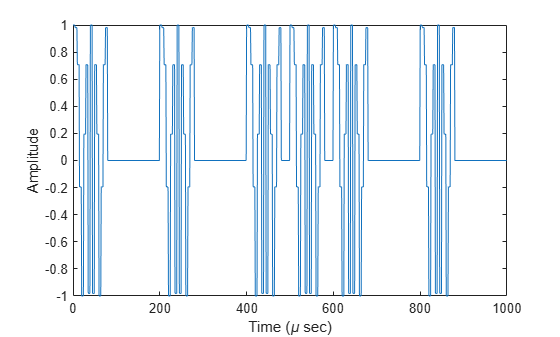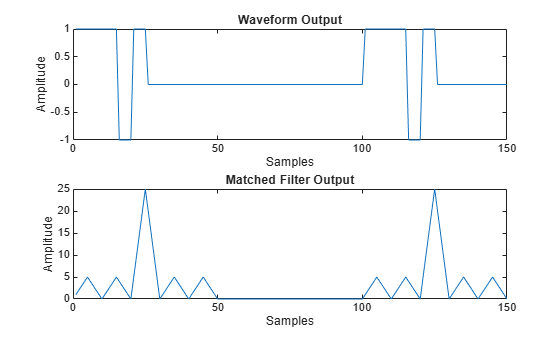# step

System object: phased.PhaseCodedWaveform
Package: phased

Samples of phase-coded waveform

## Syntax

Y = step(sPCW) Y = step(sPCW,prfidx) Y = step(sRFM,freqoffset) [Y,PRF] = step(___) [Y,COEFF] = step(___) 

## Description

Note

Starting in R2016b, instead of using the step method to perform the operation defined by the System object™, you can call the object with arguments, as if it were a function. For example, y = step(obj,x) and y = obj(x) perform equivalent operations. When the only argument to the step method is the System object itself, replace y = step(obj) by y = obj().

Y = step(sPCW) returns samples of the phase-coded pulse in a column vector, Y.

Y = step(sPCW,prfidx), uses the prfidx index to select the PRF from the predefined vector of values specified by in the PRF property. This syntax applies when you set the PRFSelectionInputPort property to true.

Y = step(sRFM,freqoffset), uses the freqoffset to generate the waveform with an offset as specified at step time. Use this syntax for cases where the transmit pulse frequency needs to be dynamically updated. This syntax applies when you set the FrequencyOffsetSource property to 'Input port'.

[Y,PRF] = step(___) also returns the current pulse repetition frequency, PRF. To enable this syntax, set the PRFOutputPort property to true and set the OutputFormat property to 'Pulses'.

[Y,COEFF] = step(___) returns the matched filter coefficients, COEFF, for the current pulse. To enable this syntax, set CoefficientsOutputPort to true. COEFF is returned as an NZ-by-1 vector, where NZ is the maximum of the nonzero pulse width.

You can combine optional input and output arguments when their enabling properties are set. Optional inputs and outputs must be listed in the same order as the order of the enabling properties. For example, [Y,PRF,COEFF] = step(sRFM,prfidx,freqoffset).

Note

The object performs an initialization the first time the object is executed. This initialization locks nontunable properties and input specifications, such as dimensions, complexity, and data type of the input data. If you change a nontunable property or an input specification, the System object issues an error. To change nontunable properties or inputs, you must first call the release method to unlock the object.

## Input Arguments

 sPCW Phase-coded waveform object.

## Output Arguments

 Y Column vector containing the waveform samples.

## Examples

expand all

Generate samples of two pulses of a phase-coded pulse waveform that uses the Zadoff-Chu code.

sPCW = phased.PhaseCodedWaveform('Code','Zadoff-Chu',... 'ChipWidth',1e-6,'NumChips',16,... 'OutputFormat','Pulses','NumPulses',2); wav = step(sPCW); fs = sPCW.SampleRate; nsamps = size(wav,1); t = [0:(nsamps-1)]/fs; plot(t*1e6,real(wav)) title('Waveform: Real Part') xlabel('Time (\mu sec)') ylabel('Amplitude') gridCreate and plot two-pulse phase-coded waveforms that uses the Zadoff-Chu code. Set the sample rate to 1 MHz, a chip width of 5 microseconds, 16 chips per pulse. Vary the pulse repetition frequency.

fs = 1e6; PRF = [5000,10000]; waveform = phased.PhaseCodedWaveform('SampleRate',fs,... 'Code','Zadoff-Chu','PRFSelectionInputPort',true,... 'ChipWidth',5e-6,'NumChips',16,'PRF',PRF,... 'OutputFormat','Pulses','NumPulses',2);

Obtain and plot the phase-coded waveforms. For the first call to the step method, set the PRF to 10kHz using the PRF index. For the next call, set the PRF to 25 kHz. For the final call, set the PRF to 10kHz.

wav = []; wav1 = waveform(1); wav = [wav; wav1]; wav1 = waveform(2); wav = [wav; wav1]; wav1 = waveform(1); wav = [wav; wav1]; nsamps = size(wav,1); t = [0:(nsamps-1)]/fs; plot(t*1e6,real(wav)) xlabel('Time (\mu sec)') ylabel('Amplitude')Generate output samples and matched filter coefficients of a Barker coded waveform.

waveform = phased.PhaseCodedWaveform('Code','Barker','NumChips',5, ... 'CoefficientsOutputPort',true,'PRF',[1e4 2e4],'ChipWidth',5e-6, ... 'OutputFormat','Samples','NumSamples',150); [wav,coeff] = waveform();

Create a matched filter that applies the coefficients as an input argument. Use the coefficients when applying the matched filter to the waveform. Plot the waveform and matched filter outputs.

mf = phased.MatchedFilter('CoefficientsSource','Input port'); mfOut = mf(wav,coeff); subplot(211),plot(real(wav)); xlabel('Samples'),ylabel('Amplitude'),title('Waveform Output'); subplot(212),plot(abs(mfOut)); xlabel('Samples'),ylabel('Amplitude'),title('Matched Filter Output');